# Homework H.2.I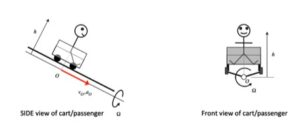# Homework H.2.JDiscussionThe motion of this four-bar mechanism is quite complicated. However, we can make some sense out of the velocities of points B and D by using instant centers (ICs). For a given position of the mechanism, can you visualize the location of the IC for link BD? And from this location, do the directions for the velocities for points B and D make sense?

HINTS:
You are asked to perform the complete kinematical analysis for the mechanism for the position shown below.

Velocity
For the velocity analysis, you are encouraged to use the IC center approach. Where is the IC for link BD located? What does the location of this IC say about the angular velocity of link BD? Use that result to determine the angular velocity of link DE.

Acceleration
This is a standard kinematics of a mechanism problem. For this, consider the following steps:

1. Write down the acceleration of point B using link AB.
2. Write down the acceleration expression for D using link DE.
3. Write down the acceleration expression for B using link DB and your result for the acceleration of D from Step 2.
4. Equate the expressions for the acceleration of B in Steps 1 and 3 above. From this, solve for the angular accelerations of links BD and DE.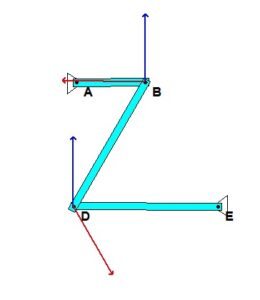# Homework H.2.GDiscussion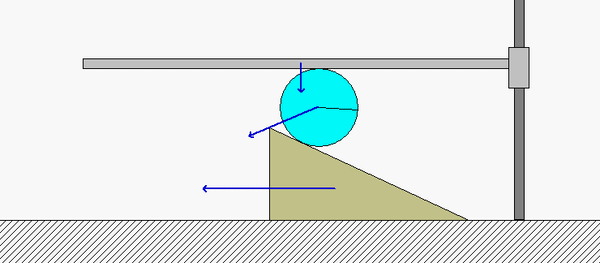Points B and D on the disk roll without slipping on the horizontal guide A and the wedge E, respectively. Because of this:

• B has a velocity vB which points straight down, with the same speed vA as the horizontal guide A.
• D has a velocity vD which points directly to the left with the same speed as the wedge E.

The instant center (IC) of the disk (call it point C) therefore exists at the intersection of the perpendiculars to vand vD. Note that point B is much closer to the IC than point D. What does this say about the relative sizes of vD and vB? Is this consistent with the speeds of D and B shown in the above animation?

HINTS:

• Use the above discussion points to locate the IC for the disk.
• Determine the angular speed of the disk based on the speed of B and the distance from B to C.
• The speed of the wedge E is found by using the angular speed from above and the distance from C to D.

# Homework H.2.HDiscussion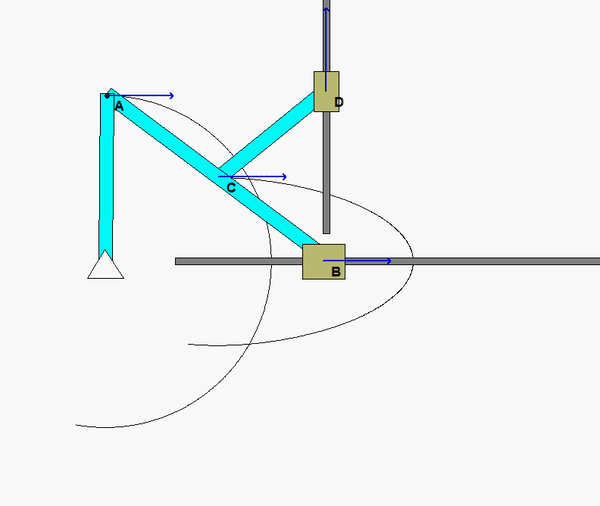The animation above shows the motion of the mechanism over a range of input angles of link OA. For a given position, envision the location of the instant centers (ICs) for links AB and CD. Do the directions and magnitudes for the velocities of points B, C and D agree with the location of these ICs?

Shown below if a freeze-frame of the mechanism motion at the position for which you are asked to do analysis. From this figure, where are the two ICs for links CD and BC? In particular, how does the position of the IC for AB relate to the relative sizes of the speeds of points A, C and B? What is the angular velocity of link AB at this position?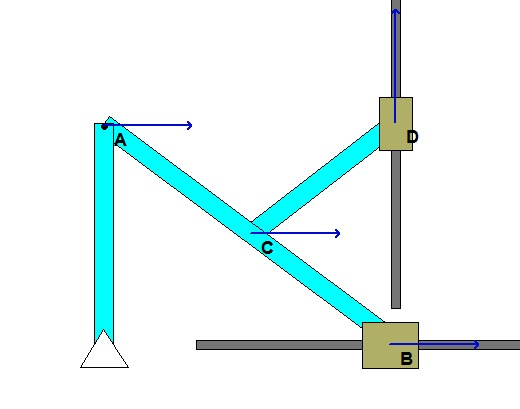# Homework H.2.E# Homework H.2.FDiscussionAs you plan your solution, consider that for the velocity part of the problem, you will need to use a velocity equation for bar AB and two velocity equations for the disk:

• Write the velocity of point B referenced to point A on bar AB.
• Write the velocity of point O referenced to the contact point C on the disk. Note that since the disk does not slip, the velocity of point C is zero.
• Write the velocity of point B referenced to point O on the disk.

These equations together will provide you with the equations that you need for the angular velocities of the bar and disk.

Repeat this same process above for accelerations. Please note that the acceleration of C on the disk is NOT zero - instead, you know that its acceleration is strictly in the y-direction.

# Homework H.2.C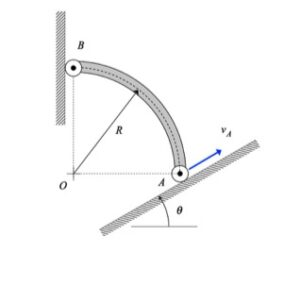Discussion and hints:

The solution for the velocity and acceleration of end B is a straight-forward application of the rigid body velocity and acceleration equations for member AB:

vB = vA + omega x rB/A
aB = aA + α x rB/A - ω2*rB/A

where vB = vBj_hat, aB = aB*j_hat and aA = aA*(cos(θ)*i_hat + sin(θ)*j_hat)Each of the two vector equations above represents two scalar equations, providing us with the necessary equations to solve for vB, ω, aB and α. All of the observations made above can be predicted by the above kinematics equations. Instant centers (later on in the course) can prove useful in providing explanations.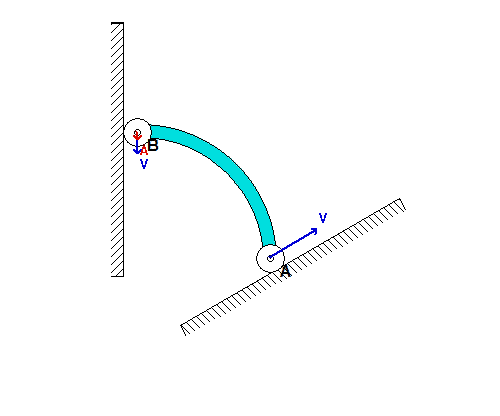For the inclination angle used in the above simulation, we see that point B moves DOWNWARD along the vertical wall as A moves up along the incline. As B moves onto the same horizontal plane as A, the acceleration of B becomes very large (although A continues to move with a constant speed). Can you provide a physical explanation for this?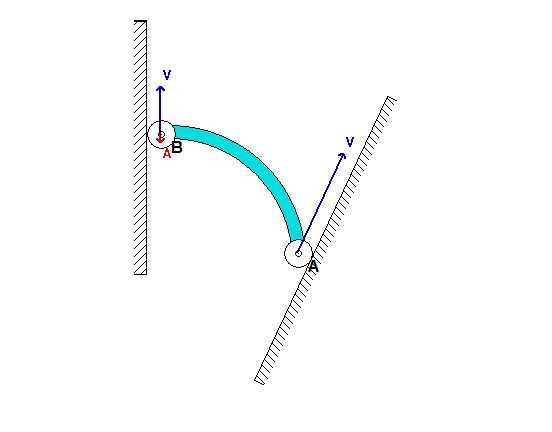If we now consider a steeper inclination angle for A, as used above, we see that end B initially moves UPWARD along the wall; however, at some point B reverses its direction and begins to move DOWNWARD along the wall. Can you provide a physical explanation for this? Note also that the acceleration of B becomes very large as B moves onto the same horizontal plane as A, as it was for the initial value of inclination angle.

What is the value of the incline angle theta that defines the boundary between the types of initial motions for bar AB shown in the above two simulations? For the numerical value of the angle theta provided in the problem statement, which of the two simulations above agree with your results?

# Homework H.2.DAnimation of motion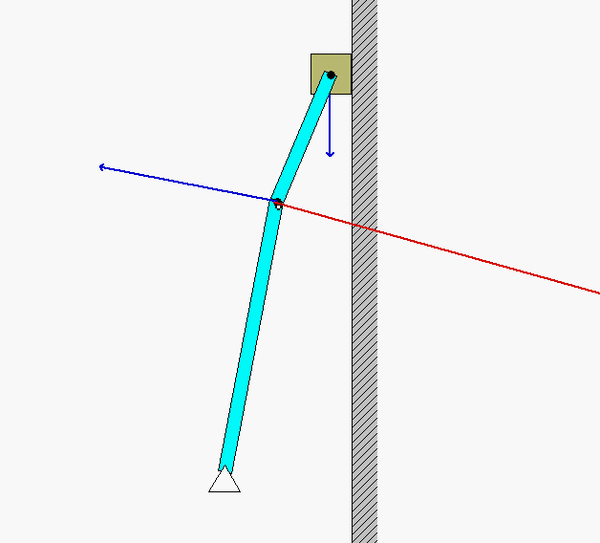Note that as link BD passes through the horizontal orientation, the velocity of B (as well as the angular velocity of AB) instantaneously goes to zero, yet the acceleration of B is not zero. In a couple days when we cover instant centers of zero velocity we will be able to predict when point B has zero velocity.

Solution plan

1. Note that since D is traveling on a straight line with constant speed, the acceleration of D is zero. Therefore, we can write: a_B = a_D + alpha_BD x r_B/D - omega_BD^2*r_B/D = alpha_BD x r_B/D - omega_BD^2*r_B/D.
2. Note that since A is a pin joint, the acceleration of A is zero. Therefore, we can write: a_B = a_A + alpha_AB x r_B/A - omega_AB^2*r_B/A = alpha_AB x r_B/A - omega_AB^2*r_B/A.
3. Equate the expressions of a_B  from 1. and 2. above. This gives you two SCALAR equations in order to solve for two unknowns, alpha_AB and alpha_BD.

Note that you need to solve the velocity problem first. Follow the above procedure to do so. Here. the velocity of D is known as v_D = -v_D*j_hat.

# Homework H.2.ADiscussionNote that the plate rotates about point O. Therefore, O is the center of the circular paths of points A and B. From the animation above, we see that the velocities of A and B are tangent to their circular paths, as expected. The accelerations of A and B are NOT perpendicular to the paths of A and B since the speeds of A and B are increasing in time (and consequently, A and B each have positive tangential components of acceleration).

Initially, the acceleration for these two points is nearly aligned with velocity, since the speeds are small and therefore the centripetal components of acceleration are small. Near the end of the first revolution of the plate, the speeds have increased to the point where the centripetal components of acceleration dominate, and acceleration is nearly perpendicular to the path.

Solution hints
For Part a) of this problem, it is recommended that you use the rigid body kinematics equations using point O as the reference point, since the velocity and acceleration of O are zero. That is, you should use v_B = v_O + Ω x r_B/O and a_B = a_O + Ω_dot x r_B/O - Ω^2*r_B/O. Repeat the process for finding the velocity and acceleration of A.

For Part b) of this problem, it is recommended that you use the rigid body kinematics equations with point A first. This will give you the equations needed to find Ω_dot. Then, use the rigid body kinematics equations to find the acceleration of B.

# Homework H.2.B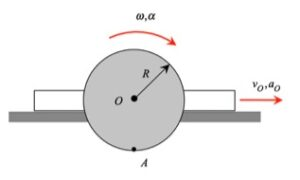DiscussionThe velocity of A is always tangent to the path of A, and the acceleration of A always points inward on the path of A, as expected.

In solving the problem, write the velocity and acceleration of A referenced to the motion of the center of disk, O. Point O travels on a straight path, so its velocity and acceleration are always in the horizontal direction. Note that for a standard xy-coordinate system with x positive to the right and y positive upward, the angular velocity and angular acceleration of the disk are in the -z direction.